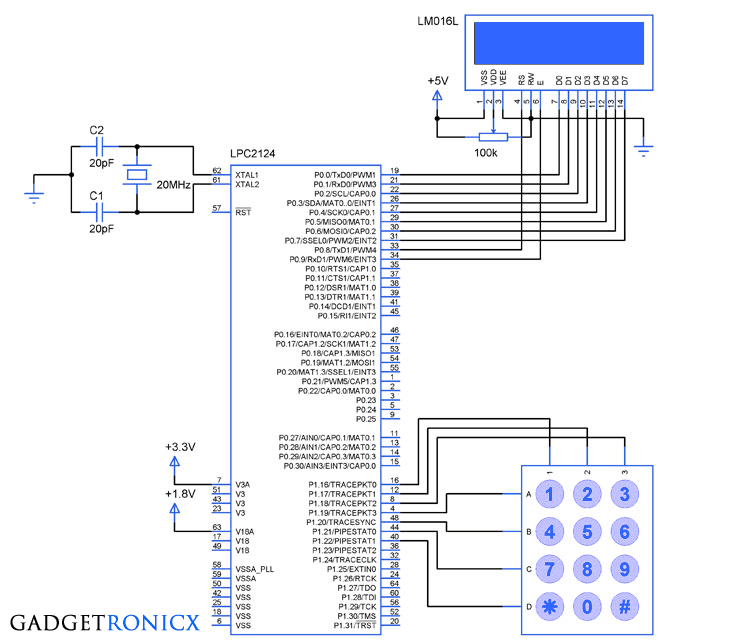# Keypad and LCD interfacing with ARM7 MIcrocontrollerKeypad interface with ARM7 Microcontroller

Keypads are the most commonly used input device in many embedded system since it possess simple design and also comes at affordable cost. This makes the concept of keypad interfacing with a Microcontroller very important. We are about to see the tutorial of keypad and lcd interfacing with the ARM7 Microcontroller ( LPC2124).

The above design uses a 4×3 keypad to take input and a 16×2 LCD to display the input value fed by the keypad. If you are not familiar with LCD interfacing with ARM controller kindly go through this tutorial  “16×2 LCD interface with ARM7 Microcontroller”.

## POLLING:

Keypad interfacing can be done with the Microcontroller by means polling and interrupts. Since interrupts will not fit when interfacing large number of keys so polling becomes the ultimate choice. Polling is nothing but making the rows of the keypad as input and column as output or vice versa.

In this method each row will be made low one at a time and the corresponding logic transition in column value will be monitored by the Microcontroller. So for example if key ‘5’ pressed at any moment the Microcontroller will realize this when row 2 and column 2 is low at same instant. Note that you can monitor either row or column with the Microcontroller for logic transition.The above diagram shows the construction of a simple keypad using push buttons. Whereas i have used a ready made 4×3 keypad in the above design, you can opt for any of it. You can also expand the keypad configuration by adding more switches to it.

## CODE:

The below code was built using Keil uVision 4 and built in such a way to scan the keypad and display the input values in the LCD connected to the Controller.

```#include<lpc21xx.h>
void delay(void);
void lcd(unsigned char,int b);
char c;
int main(void)
{
PINSEL0=PINSEL2=0;
IODIR0=0x000003ff;
IODIR1=0x00780000;
lcd(0x38,0);
lcd(0x0f,0);
while(1)
{
lcd(c,1);
}
}

void lcd(unsigned char a,int b)         //LCD Subroutine
{
IOSET0=a<<0;
IOSET0=b<<8;
IOSET0=1<<9;
delay();
IOCLR0=1<<9;
IOCLR0=b<<8;
IOCLR0=a<<0;
}

{
while(1)
{
IOCLR1|=(1<<19);               //Making row1 LOW
IOSET1|=(1<<20)|(1<<21)|(1<<22); //Making rest of the rows '1'
if(!(IOPIN1&(1<<16)))             //Scan for key press
{
while(!(IOPIN1&(1<<16)));
return '1';                           //Returning value to display
}
if(!(IOPIN1&(1<<17)))
{
while(!(IOPIN1&(1<<17)));
return '2';
}
if(!(IOPIN1&(1<<18)))
{
while(!(IOPIN1&(1<<18)));
return '3';
}

IOCLR1|=(1<<20);
IOSET1|=(1<<21)|(1<<22)|(1<<19);
if(!(IOPIN1&(1<<16)))
{
while(!(IOPIN1&(1<<16)));
return '4';
}
if(!(IOPIN1&(1<<17)))
{
while(!(IOPIN1&(1<<17)));
return '5';
}
if(!(IOPIN1&(1<<18)))
{
while(!(IOPIN1&(1<<18)));
return '6';
}

IOCLR1|=(1<<21);
IOSET1|=(1<<22)|(1<<20)|(1<<19);
if(!(IOPIN1&(1<<16)))
{
while(!(IOPIN1&(1<<16)));
return '7';
}
if(!(IOPIN1&(1<<17)))
{
while(!(IOPIN1&(1<<17)));
return '8';
}
if(!(IOPIN1&(1<<18)))
{
while(!(IOPIN1&(1<<18)));
return '9';
}

IOCLR1|=(1<<22);
IOSET1|=(1<<19)|(1<<20)|(1<<21);
if(!(IOPIN1&(1<<16)))
{
while(!(IOPIN1&(1<<16)));
return '*';
}
if(!(IOPIN1&(1<<17)))
{
while(!(IOPIN1&(1<<17)));
return '0';
}
if(!(IOPIN1&(1<<18)))
{
while(!(IOPIN1&(1<<18)));
return '#';
}
}
}

void delay(void)                                    //Delay loop
{
unsigned int i;
for(i=0;i<=20000;i++);
}```

Like this article? Why not share it with others through social sites. We will post more of these ARM tutorials here , Follow/Subscribe to let us deliver these articles directly into your inbox/ timeline. Have queries? Feel free to post it below, we are happy to help you.

## JLCPCB - Only \$2 for PCB Prototype (Any Color)

Up to \$20 shipping discount on first order now: https://jlcpcb.com/quote0 Followers

Most reacted comment
3 Comment authorsRecent comment authors
Subscribe
Notify ofGuest
kumar

Hi Donald, Informative post on keypad. Nice schematics.
May I know which software you have used for design of your circuits above.wpDiscuz